### Home > CALC > Chapter 4 > Lesson 4.1.3 > Problem4-35

4-35.
1. Given f(x) = sin x, g(x) = x2 and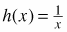, use compositions to express each of the following functions. Homework Help ✎

1. y = sin(x2)

2. y = sin2(x)

3. y = csc(x)

4.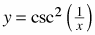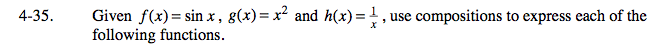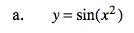x² is the inner function. sin(x) is the outer function.

y = f(g(x))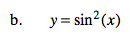x² is the outer function. What is the inner function?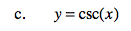Recall that y = csc(x) is the reciprocal of y = sin(x).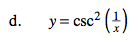Refer to the hint in part (c).